# How to Calculate and Solve for the Mass, Volume and Mass Concentration in Chemistry | The Calculator Encyclopedia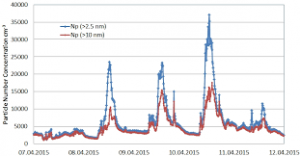The image above represents the mass concentration.

To compute the mass concentration, two essential parameters are needed and these parameters are mass (m) and volume (V).

The formula for calculating mass concentration:

ρ = mV

Where;
ρ = Mass concentration
m = Mass
V = Volume

Let’s solve an example;
Find the mass concentration when the mass is 8 kg with a volume of 24 dm³.

This implies that;
m = Mass = 8
V = Volume = 24

ρ = mV
ρ = 824
ρ = 0.33

Therefore, the mass concentration is 0.33 Kg/dm³.

Calculating the Mass when the Mass Concentration and the Volume is Given.

m = Vρ

Where;
m = Mass
ρ = Mass concentration
V = Volume

Let’s solve an example;
Find the mass when the mass concentration is 12 kg/dm³ with a volume of 7 dm³.

This implies that;
ρ = Mass concentration = 12
V = Volume = 7

m = Vρ
m = 12 x 7
m = 84

Therefore, the mass concentration is 84 kg.

Calculating the Volume when the Mass Concentration and the Mass is Given.

V = m / ρ

Where;
V = Volume
m = Mass
ρ = Mass concentration

Let’s solve an example;
Find the volume when the mass concentration is 18 kg/dm³ with a mass of 32 kg.

This implies that;
ρ = Mass concentration = 18
m  = Mass = 32

V = m / ρ
V = 32 / 18
V = 1.77

Therefore, the volume is 1.77 dm3.

Nickzom Calculator – The Calculator Encyclopedia is capable of calculating the mass concentration.

To get the answer and workings of the mass concentration using the Nickzom Calculator – The Calculator Encyclopedia. First, you need to obtain the app.

You can get this app via any of these means:

To get access to the professional version via web, you need to register and subscribe for NGN 1,500 per annum to have utter access to all functionalities.
You can also try the demo version via https://www.nickzom.org/calculator

Once, you have obtained the calculator encyclopedia app, proceed to the Calculator Map, then click on Basic Chemistry under Chemistry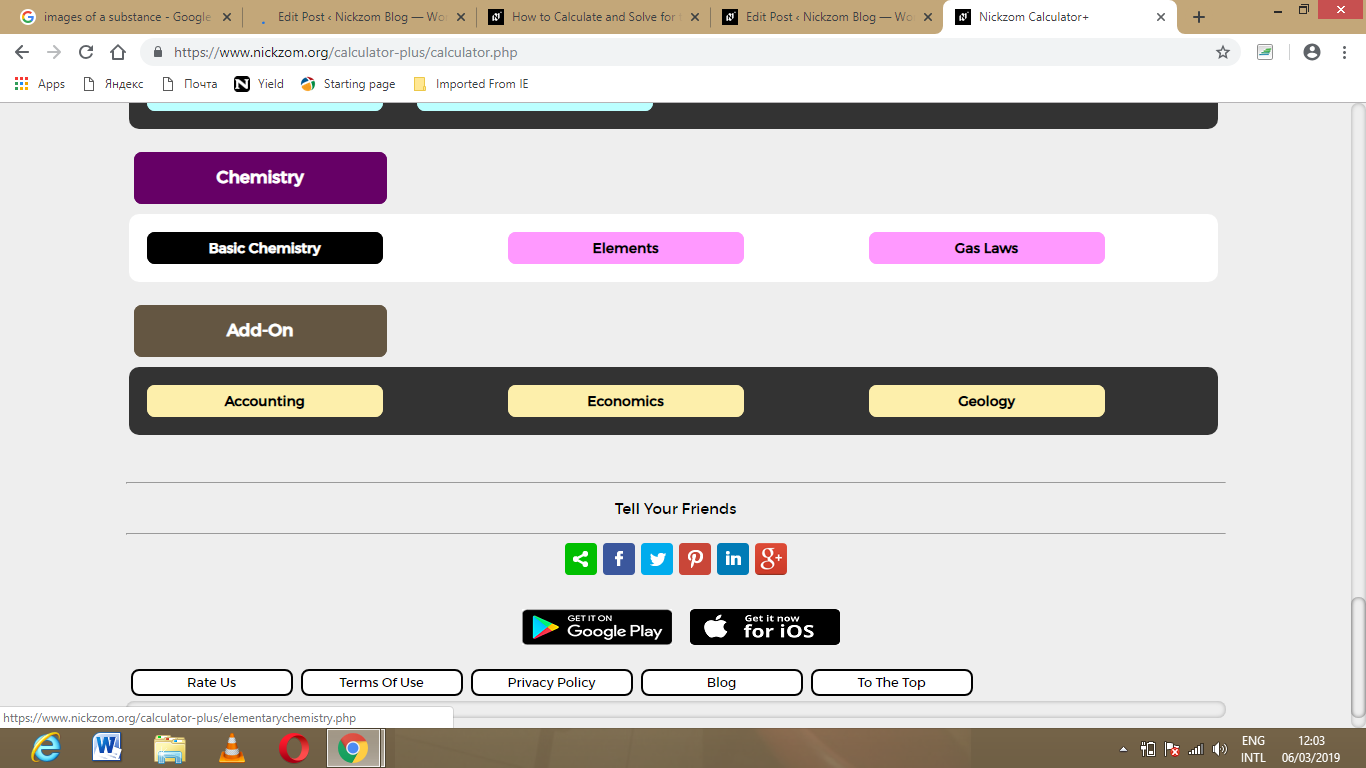Now, Click on Mass Concentration under Basic Chemistry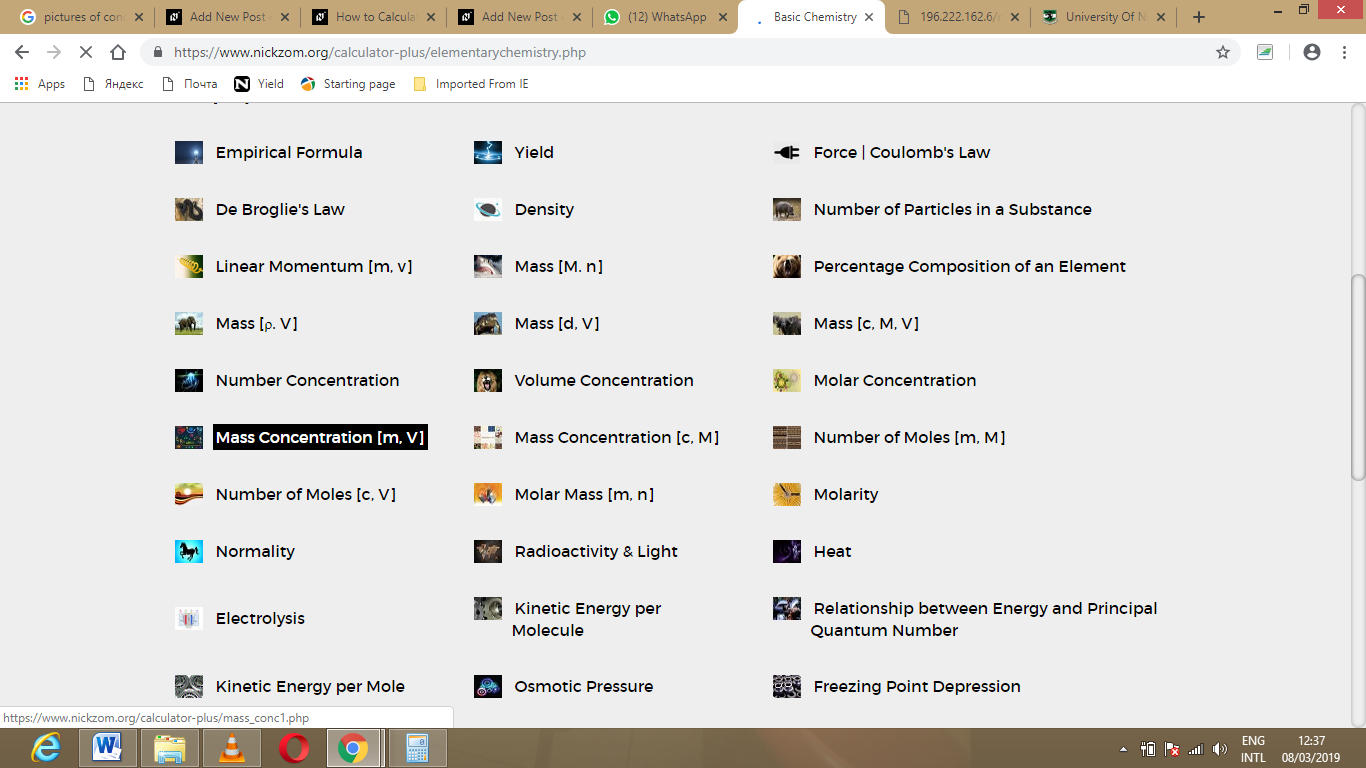The screenshot below displays the page or activity to enter your values, to get the answer for the mass concentration according to the respective parameters which are the mass (m) and volume (V).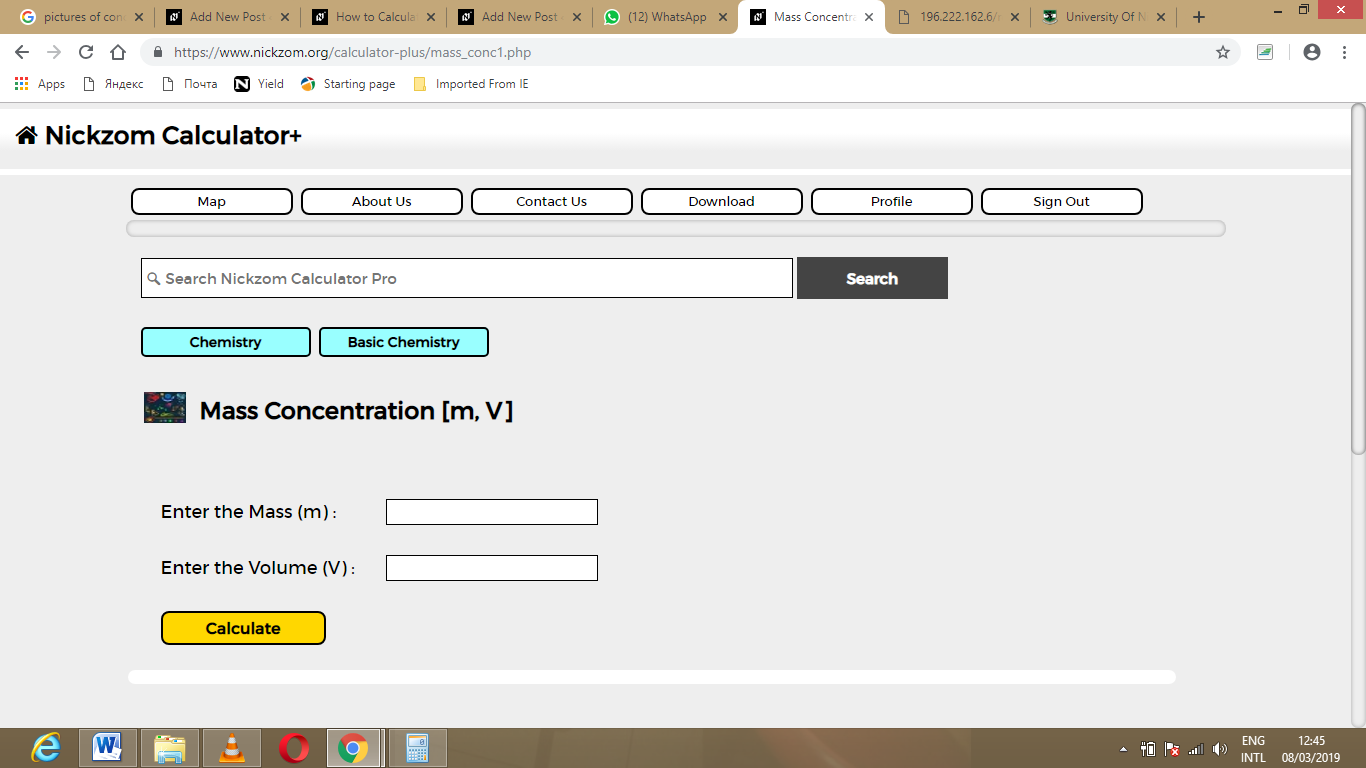Now, enter the values appropriately and accordingly for the parameters as required by the example above where the mass (m) is 8 kg and volume (V) is 24 dm3.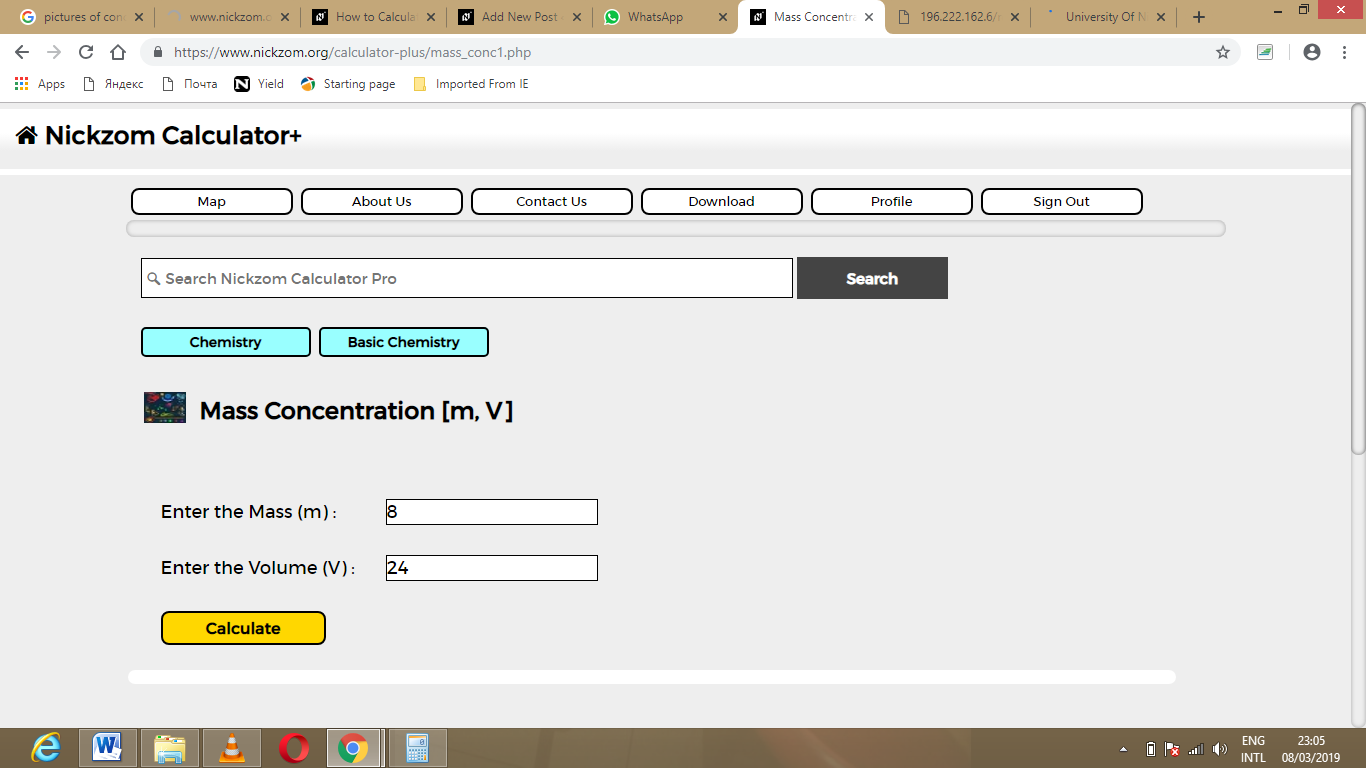Finally, Click on Calculate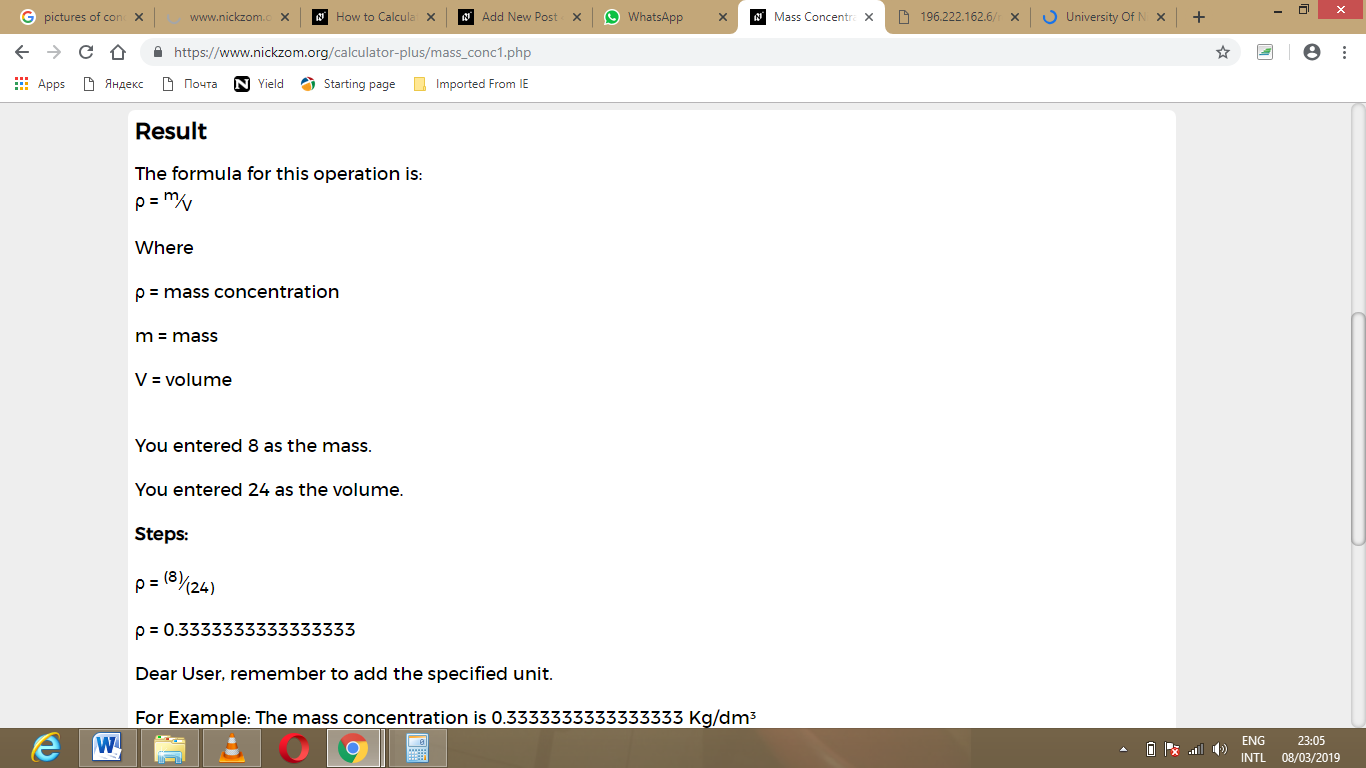As you can see from the screenshot above, Nickzom Calculator – The Calculator Encyclopedia solves for the mass concentration and presents the formula, workings and steps too.